Next: Error Analysis of Up: Gaussian Elimination (GE) Previous: Gaussian Elimination (GE)

## LU Decomposition

GE computes an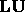factorization of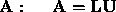, where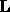is unit lower triangular and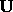is upper triangular.

Theorem: There exists a uniquefactorization of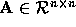if and only if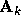is non-singular for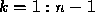. Ifis singular for some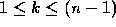then the factorization may exist, but if so it is not unique.

Proof: By induction (from Golub and Van Loan, 1992).

The effect of partial or complete pivoting is equivalent to multiplying the matrix by a permuation matrix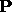. GE with partial pivoting (GEPP) applied tois equivalent to GE without pivoting applied to the row-permuted matrix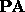. This holds for all n: GEPP computes a factorization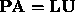.

Computing anfactorization is equivalent to solving the equations: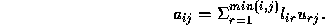If these non-linear equations are examined in the right order, they can be easily solved. For added generality let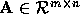,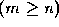, and consider anfactorization with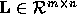and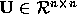(is lower triangular). Suppose we know the first k - 1 columns ofand the first k - 1 rows of. Setting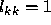,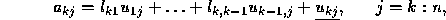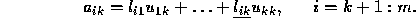We can solve for the underlined elements in the kth row ofand then the kth column of. This process is called Doolittle's method. It computes thefactorization, assuming that the factorization exists.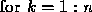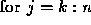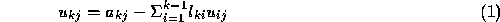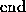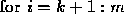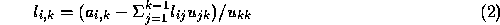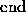Cost: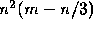flops.

Doolittle's method is mathematically equivalent to GE without pivoting, for we have,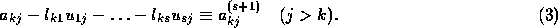Doolittle's method is well suited to calculations by hand or with a desk calculator, because it obviates the need to store the intermediate quantities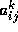. It is also attractive when we can accumulate inner products in extended precision. It is straightforward to incorporate partial pivoting into Doolittle's method. However, complete pivoting cannot be incorporated without changing the method.Next: Error Analysis of Up: Gaussian Elimination (GE) Previous: Gaussian Elimination (GE)

Dinesh Manocha
Thu Jan 29 05:58:31 EST 1998